# Homework Given January 23, 2007

## Due January 30, 2007

This assignment is due on February 6, 2007.  Chapter 1 exercises are on pages 28 through 36.

## Live Links to the Exercises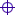Exercise 1.1Exercise 1.3Exercise 1.7

## Exercise 1.1

Use Kirchhoff's Voltage Law in Figure 1.1, page 2, to find an expression for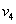in terms of the other voltages in the circuit.

The figure shows a voltage source,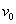, across Node X and Node Z that drives two voltage dividers.  Loop B in the figure includes the voltage source and the voltage divider involving.  The loop equation for Loop A is given in the text as Equation (1.2),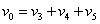.

We solve this equation for,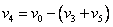.

## Exercise 1.3

Consider the circuit of Figure P1.3.  Write a set of KVL and KCL equations and use them to find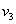.

We will resist the temptation to use Thevenin and Norton equivalent circuits to collapse the circuit from the left to find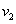and then solve the rest of the circuit to find the voltage at node 1, or to treat the parallel combination of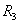and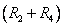with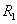as a voltage divider to find the voltage at node 1 and thence to solve the circuit, etc., because the problem statement specifically requires that we use KVL and KCL.

We will use the modified figure given below.  The values are not included with the resistor names but are given just before the numerical solution below, and signs have been placed on the resistors to show the determination of the signs to be used in designating voltage drops.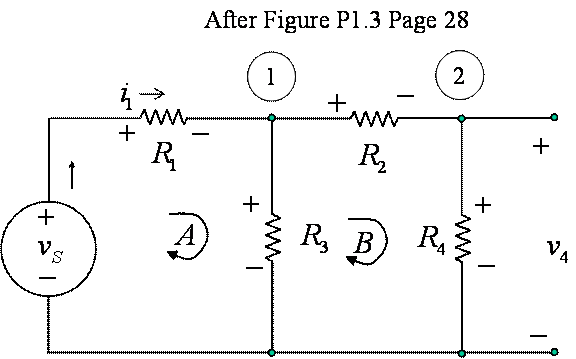Figure 1  Modified Figure P1.3 for Analysis using KVL and KCL

We will solve the circuit with a matrix equation, and give the equation for the voltage, followed by the numerical solution of the entire circuit.  We begin by writing the KVL loop equations, followed by the KCL node equations.  We use Ohm's Law to obtain equations in the currents through the resistors.  We conclude with a second look using node voltage notation.We will simplify our equations to three equations in three unknowns by noting that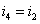:The solution can be found by Cramer's Rule (substituting the right hand side vector for the coefficients of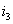and finding the determinant, then dividing by the determinant of the unmodified matrix), Gaussian elimination (adding and subtracting rows to eliminate unknowns untilis found), using the TI-89 or other software to solve the entire equation symbolically or numerically, and other methods.  Cramer's rule is particularly tempting because the right hand side vector has just one nonzero element, so evaluating the determinants by minors about that column requires that we evaluate only two by two determinants for the numerator, and the only three by three determinant is the denominator in common with all three currents.  Here we give the general solution,where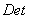is the determinant of the matrix,.

Note that the determinant cannot be zero unless bothandare zero, i.e. the source is shorted, which is consistent with the circuit as given.  The solution isso we have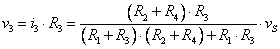.

The values in the problem statement are given in the figure in the text asso, numerically, we haveand, with Ohm's law, we have the voltages across the resistors asWe will look at the equations using node voltage notation as a check.  Repeating the loop equations,The matrix form of these equations iswhere we have used conductance, the reciprocal of resistance, to save space.  The notation is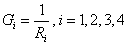.

The solution is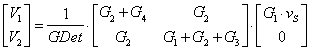where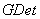is the determinant of this matrix,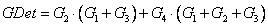.

The numerical solution is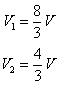which agrees with the previous solution.

## Exercise 1.7

Use KVL for the two loops in the circuit of Figure 1.65(a) to find a value for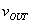.

Figure 1.65(a) is on page 33, and is part of problem 1.65 which is not assigned here.  The two loops are both counterclockwise.  The loop equations are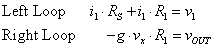Note that we usedas the voltage across the controlled current source.  Also, note that the there are two resistors denotedin the circuit of Figure 1.65(a).

Since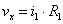, all we need is, which we can get from the first loop:,

which is seen to be a straightforward application of Ohm's law.  Combining these equations we have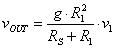.

The circuit is a simplified model of a FET transistor, and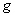is the transconductance of the transistor – the ratio of current out to voltage input, and has the physical units of conductance, or reciprocal ohms.